Linear Graphs

Chapter 15 Class 8 Introduction to Graphs
Concept wise

In linear graph, the line is straight.

There is no bend unlike line graph

## Check whether it is a linear graph or not?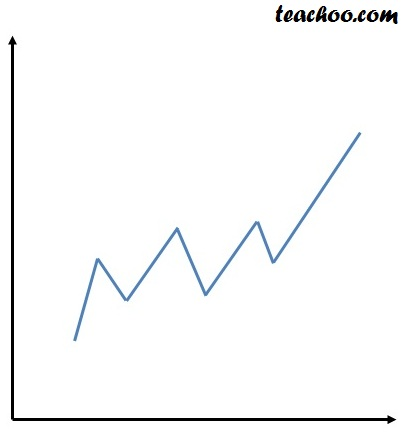-a-

Not a linear graph as line is not straight

-ea-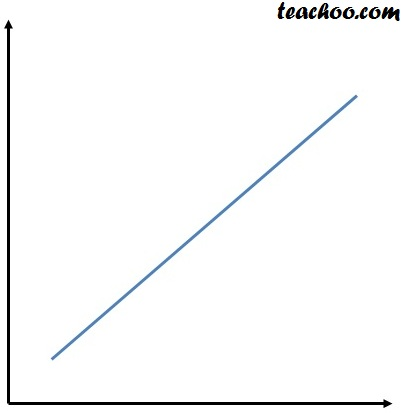-a-

Line is straight.

So, Linear graph

-ea-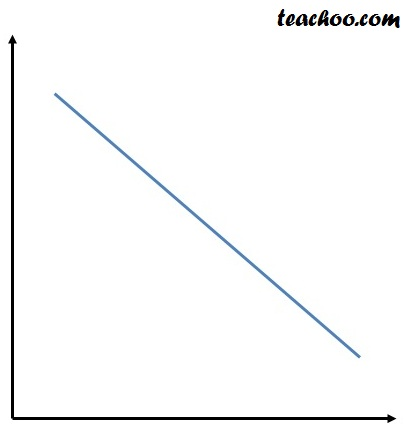-a-

Line is straight.

So, Linear graph

-ea-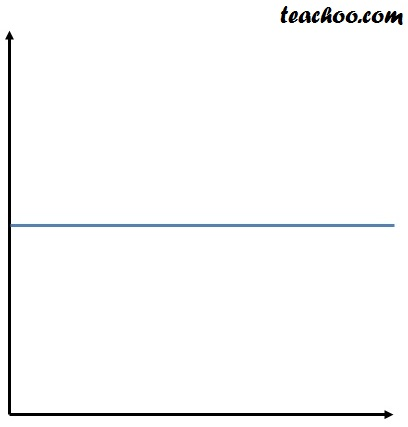-a-

Line is straight.

So, Linear graph

-ea-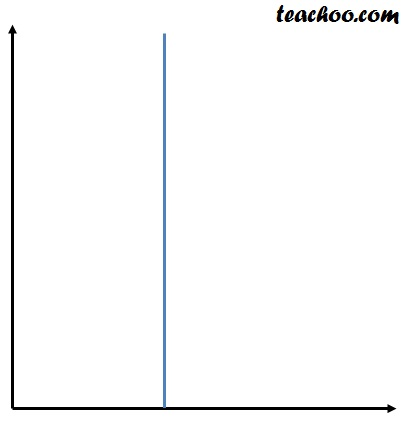-a-

Line is straight.

So, Linear graph

-ea-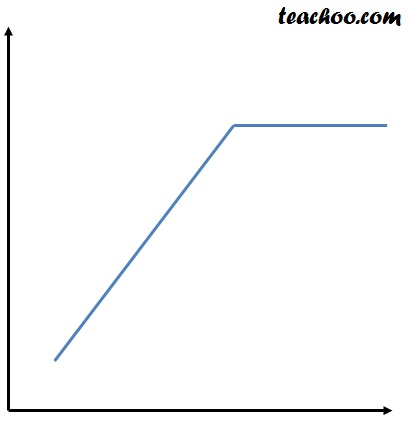-a-

Not a linear graph as line is not straight

-ea-

Get live Maths 1-on-1 Classs - Class 6 to 12